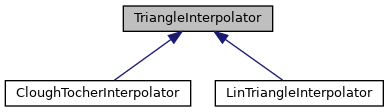TriangleInterpolator Class Referenceabstract

This is an interface for interpolator classes for triangulations. More...

`#include <TriangleInterpolator.h>`

Inheritance diagram for TriangleInterpolator:[legend]

## Public Member Functions

virtual ~TriangleInterpolator ()=default

virtual bool calcNormVec (double x, double y, QgsPoint &result)=0
Calculates the normal vector and assigns it to vec. More...

virtual bool calcPoint (double x, double y, QgsPoint &result)=0
Performs a linear interpolation in a triangle and assigns the x-,y- and z-coordinates to point. More...

## Detailed Description

This is an interface for interpolator classes for triangulations.

Note
Not available in Python bindings.

Definition at line 34 of file TriangleInterpolator.h.

## ◆ ~TriangleInterpolator()

 virtual TriangleInterpolator::~TriangleInterpolator ( )
virtualdefault

## ◆ calcNormVec()

 virtual bool TriangleInterpolator::calcNormVec ( double x, double y, QgsPoint & result )
pure virtual

Calculates the normal vector and assigns it to vec.

Implemented in CloughTocherInterpolator, and LinTriangleInterpolator.

## ◆ calcPoint()

 virtual bool TriangleInterpolator::calcPoint ( double x, double y, QgsPoint & result )
pure virtual

Performs a linear interpolation in a triangle and assigns the x-,y- and z-coordinates to point.

Implemented in CloughTocherInterpolator, and LinTriangleInterpolator.

The documentation for this class was generated from the following file: